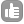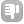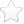0Are there tools that allow to create raw data files in vendor format that contain desired data? For example for the testing purposes one might want to write a specific value at all points. Or perhaps there is some other need to store data in the vendor format - is there software for that? asked Aug 03 '10 at 14:39Evgeny Fadeev 577●11This would be easiest for the Bruker format as there are no parameters or other information pre-pended to the data. For a single line spectrum, simply generate an exponentially decreasing cosine wave interleaved with an exponentially decreasing sine wave and save these into an array. e.g the even points would be A cos(2Pi f t) exp(-t/T2) while the odd points would be A sin(2Pi f t) exp(-t/T2) (pardon my lack of equation formatting). With A being the maximum amplitude of the fid. Simply write this array out as a file of 32bit signed integers. Make sure the value of A is reasonable for 32 bit integers. After transform, you should see a single line at frequency f of width governed by T2. Varian would be the same, but you need to pre-pend a file header and block header to the file (as discussed elsewhere in this forum). You also need to create parameter files (e.g. acqus, procpar, etc.) consistent with your synthetic fid. The cheap and dirty (i.e. easy) way to do this is create an empty data set on the spectrometer with the correct values for your fid. The parameter files are ASCII, so that you can always edit them by hand if necessary. JEOL is left as an exercise for the interested student. answered Aug 04 '10 at 11:13Kirk Marat 7111Hey Evgeny, To actually get the data to disk (for testing) the following python commands are invaluable (I find). This works well for Bruker raw data, if anyone knows the structure of Jeol and Varian files I'm sure I can accommodate. Anyway, after installing numpy for python (numerical-python), code: ``````### For a 2D [2048, 128] size spectrum >>> import numpy >>> dataSize = 2048 * 128 >>> data = numpy.zeros( dataSize, dtype=numpy.float32 ) ### Set the data to your test values (this sets all to 1.) >>> data = 1. >>> data.tofile( open( 'ser', 'wb'), '', '%f') `````` Writes the data to 'ser'. Note that the data is 32 bit float (can be 32 int) - the numpy default on modern computers is 64 bit (and Bruker data is NOT!). This also produces little endian data, and often older Bruker consoles produce big endian data. You can easily swap the endianess using 'swap' from Wayne Boucher's Azara suite. Do let me know if this was helpful! Thanks, Daniel O'Dononvan answered Aug 05 '10 at 00:08Evgeny Fadeev 577●1 Hi Dan, I think using numpy is a great idea. Thanks for bringing this up! - Evgeny Fadeev (Aug 05 '10 at 08:54)1If you want to generate Bruker data, I recommend "NMRSim" (which comes with TopSpin or even XWinNMR). This allows you to generate a dataset containing whatever you like. I find it useful when creating artificial peaks for quantitation. answered Aug 24 '10 at 06:55